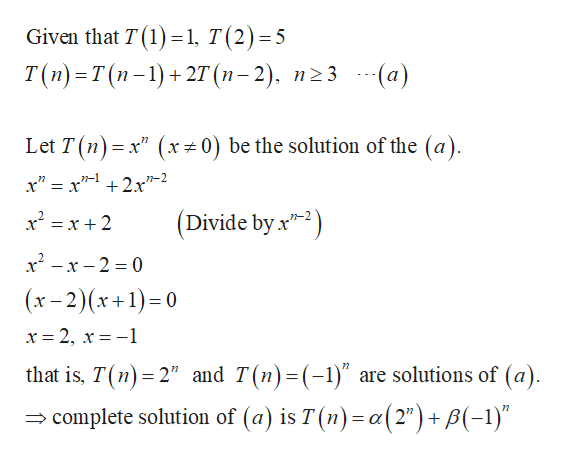# Find the closed form solution for the recurrence relation defined byT(1) = 1, T(2) = 5T(n)= T(n-1)+2T(n-2), n≥3

Question
1 views

Find the closed form solution for the recurrence relation defined by

T(1) = 1, T(2) = 5

T(n)= T(n-1)+2T(n-2), n≥3

check_circle

Step 1help_outlineImage TranscriptioncloseGiven that T (1) =1, T(2)=5 T(п) -т(п-1) + 2T (п-2). п23 -(а) %3D Let T (n) = x" (x÷0) be the solution of the (a). n-1 x" = x + 2.x"-2 x² = x + 2 (Divide by x"-2) x - x – 2 = 0 (x- 2)(x+1)= 0 x = 2, x = -1 that is, T(n) = 2" and T(n)=(-1)" are solutions of (a). = complete solution of (a) is T (n) = a(2") + B(-1)" fullscreen

### Want to see the full answer?

See Solution

#### Want to see this answer and more?

Solutions are written by subject experts who are available 24/7. Questions are typically answered within 1 hour.*

See Solution
*Response times may vary by subject and question.
Tagged in

### Math